Latest Banking jobs   »   Basic Quantitative Aptitude Quiz for All...

# Basic Quantitative Aptitude Quiz for All Banking Exams- 18th May

Q1. A train crosses a man, who is running in the same direction of train at the speed of 2m/sec. in 10 seconds. The same train crosses a tunnel in 54 seconds. If speed of train is 72 km/h then what is the length of tunnel?
(a) 850 m
(b) 800 m
(c) 900 m
(d) 750 m
(e) 650 m

Q2. Raghav can beat Suresh by 100m in a race of 1 km and they run at speed of 10m/sec and 8 m/sec respectively. If Suresh increases his speed by 7m/sec then by how much time he will beat Raghav in the same race of 1km?
(a) 43 sec
(b) 36 sec
(c) 110/3 sec
(d) 100/3 sec
(e) 70/3 sec

Q3. A boat can cover upstream distance between two points in 6 hours and same distance downstream in 4 hours. If speed of boat in still water is 8 km/h, then find speed of stream.
(a) 2 km/h
(b) 1.6 km/h
(c) 3.2 km/h
(d) 4.8 km/h
(e) 3.8 km/h

Q4. Two trains X and Y cross each other in 48 sec, when both are running in same direction. If length of train X is 160 m and speed of train X and train Y are 54 km/h and 72 km/h respectively then find the length of train Y.
(a) 160 m
(b) 120 m
(c) 80 m
(d) 100 m
(e) 95 cm

Q5. A train P running with speed 54 kmph crosses a man in 30 sec. In how much time it can cross a 180 m long platform.
(a) 51 sec
(b) 45 sec
(c) 42 sec
(d) 39 sec
(e) 58 sec

Q6. A boat can travel with the speed of 17 kmph in upstream. If the speed of river is 3 kmph, then find the speed of boat in downstream in the same river.
(a) 23 kmph
(b) 20 kmph
(c) 25 kmph
(d) 19 kmph
(e) 21 kmph

Q7. A 570 m long train can cross a pole in 38 sec. In how much time it can cross a 660 m long platform.
(a) 82 sec
(b) 64 sec
(c) 90 sec
(d) 120 sec
(e) 72 sec

Q8. Ratio between length of trains A and B is 3 : 5. Speed of train A is 72 km/h and that of train B is 54 km/h & they are running opposite to each other. If train A cross’s train B in 16 seconds then find length of train B.
(a) 350 m
(b) 250 m
(c) 450 m
(d) 150 m
(e) 320 m

Q9. Speed of a boat in still water is 8 km/h. It takes 5 hours to go upstream and 3 hours downstream distance between two points. What is the speed of stream?
(a) 4 km/h
(b) 2 km/h
(c) 3 km/h
(d) 1 km/h
(e) 2.5 km/h

Q10. Speed of a train is 90 km/h. It crosses a platform and a pole in 36 seconds and 6 seconds respectively. Find the length of platform.
(a) 450m
(b) 650 m
(c) 750m
(d) 850m
(e) 550m

Q11. A man covers half of total distance with 12 km/h and another half distance with 24km/h. Find his average speed.
(a) 12 km/h
(b) 16 km/h
(c) 10 km/h
(d) 18 km/h
(e) 6 km/h

Q12. The ratio of speed of two trains which are running in the same direction is 4 : 5. The train having higher speed crosses the second train in 30 seconds and a pole in 4 seconds respectively. Find the ratio of their lengths.
(a) 1 : 2
(b) 2 : 3
(c) 3 : 5
(d) 4 : 5
(e) 6 : 7

Q13. Speed of a boat in still water is 300% more than the speed of current. The boat takes a total time of 8 hours to cover a distance of 45 km upstream and 45 km in downstream both. Find speed of current.
(a) 2.5 km/h
(b) 2 km/h
(c) 4 km/h
(d) 3 km/h
(e) 5 km/h

Q14. Two busses start from Delhi to Karnal at 8 am and 10 am respectively. Speed of bus starting at 8 a.m. and speed of bus starting at 10 a.m. is 20 m/sec and 25 m/sec respectively. If distance between Delhi to Karnal is 200 km then at what time faster bus will catch the slower bus?
(a) 8h
(b)10 h
(c) 12 h
(d) 6 h
(e) 14 h

Q15. Ratio of the speeds of Ajay and Ramesh is 2 : 5. If Ajay covers 240 km in 8hrs then in how much time Ramesh will cover 780 km of distance?
(a) 10hrs 24minutes
(b) 5 hrs 48 minutes
(c) 8 hrs 40minutes
(d) 12 hrs 20 minutes
(e) 10 hrs and 36 minutes

Practice More Questions of Quantitative Aptitude for Competitive Exams:

Solutions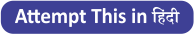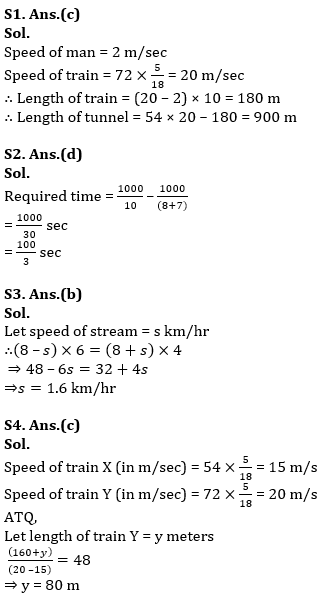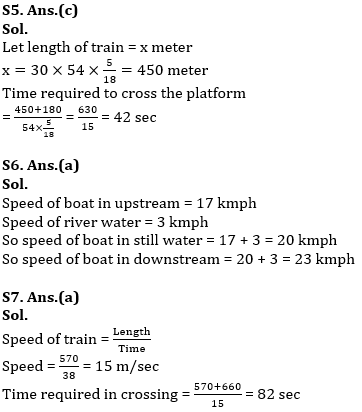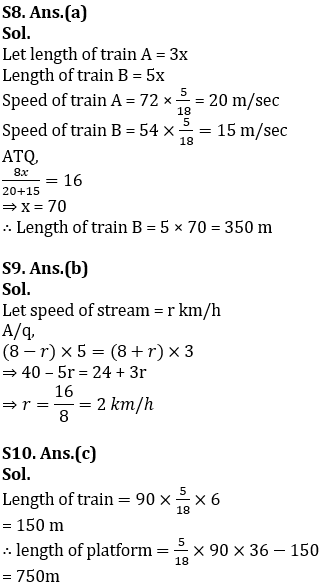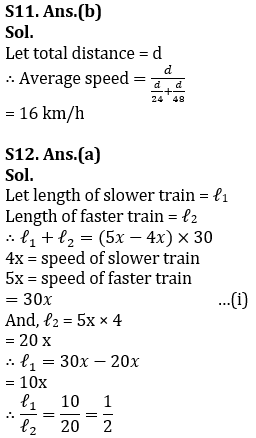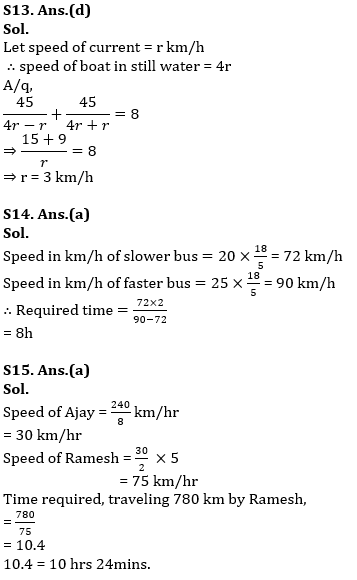#### Congratulations!Incorrect details? Fill the form again here

•Reasoning Ability Quiz For IBPS RRB PO C...
•Quantitative Aptitude Quiz For IBPS RRB ...
•Important Current Affairs Quiz for Bank ...
•Reasoning Ability Quiz For IBPS Clerk Pr...
•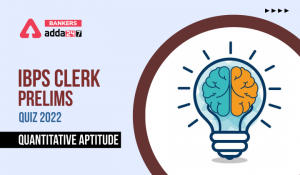Quantitative Aptitude Quiz For IBPS Cler...
•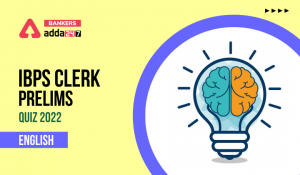English Quizzes For IBPS Clerk Prelims 2...